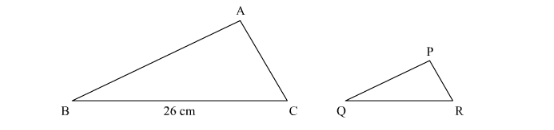# The areas of two similar triangles are 169 cm2 and 121 cm2 respectively.Question:

The areas of two similar triangles are $169 \mathrm{~cm}^{2}$ and $121 \mathrm{~cm}^{2}$ respectively. If the longest side of the larger triangle is $26 \mathrm{~cm}$, what is the length of the longest side of the smaller triangle?

Solution:

Let $\triangle \mathrm{ABC}$ and $\triangle \mathrm{PQR}$ are similar triangles. The area of triangles is $169 \mathrm{~cm}^{2}$ and $121 \mathrm{~cm}^{2}$, respectively.

Longest side of the larger triangle is 26cmTO FIND: length of longest side of the smaller side.

Suppose longest side of the larger triangle is BC and longest side of the smaller triangle is QR.

We know that the ratio of the areas of two similar triangles is equal to the ratio of the squares of any two corresponding sides.

Hence,

$\frac{a r(\Delta \mathrm{ABC})}{a r(\Delta \mathrm{PQR})}=\frac{\mathrm{BC}^{2}}{\mathrm{QR}^{2}}$

$\frac{169}{121}=\frac{26^{2}}{\mathrm{QR}^{2}}$

$\frac{13}{11}=\frac{26}{Q R}$

$\mathrm{QR}=\frac{11 \times 26}{13}$

$Q R=22$

$Q R=22 \mathrm{~cm}$